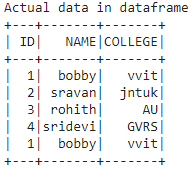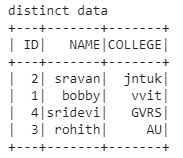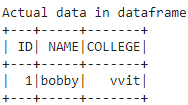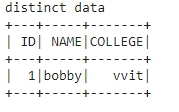# How to get distinct rows in dataframe using PySpark?

• Last Updated : 30 May, 2021

In this article we are going to get the distinct data from pyspark dataframe in Python, So we are going to create the dataframe using a nested list and get the distinct data.

We are going to create a dataframe from pyspark list bypassing the list to the createDataFrame() method from pyspark, then by using distinct() function we will get the distinct rows from the dataframe.

Syntax: dataframe.distinct()

Where dataframe is the dataframe name created from the nested lists using pyspark

Example 1: Python code to get the distinct data from college data in a data frame created by list of lists.

## Python3

 `# importing module ` `import` `pyspark ` ` `  `# importing sparksession from  ` `# pyspark.sql module ` `from` `pyspark.sql ``import` `SparkSession ` ` `  `# creating sparksession and giving ` `# an app name ` `spark ``=` `SparkSession.builder.appName(``'sparkdf'``).getOrCreate() ` ` `  `# list  of college data ` `data ``=` `[[``"1"``, ``"bobby"``, ``"vvit"``],  ` `        ``[``"2"``, ``"sravan"``, ``"jntuk"``], ` `        ``[``"3"``, ``"rohith"``, ``"AU"``], ` `        ``[``"4"``, ``"sridevi"``, ``"GVRS"``],  ` `        ``[``"1"``, ``"bobby"``, ``"vvit"``]] ` ` `  `# specify column names ` `columns ``=` `[``'ID'``, ``'NAME'``, ``'COLLEGE'``] ` ` `  `# creating a dataframe from the  ` `# lists of data ` `dataframe ``=` `spark.createDataFrame(data, columns) ` ` `  `print``(``'Actual data in dataframe'``) ` `dataframe.show() `

Output:Now Get the distinct rows in dataframe:

## Python3

 `print``(``'distinct data'``) ` ` `  `# display distinct data ` `dataframe.distinct().show() `

Output:Example 2: Python program to find distinct values from 1 row

## Python3

 `# importing module ` `import` `pyspark ` ` `  `# importing sparksession from  ` `# pyspark.sql module ` `from` `pyspark.sql ``import` `SparkSession ` ` `  `# creating sparksession and giving ` `# an app name ` `spark ``=` `SparkSession.builder.appName(``'sparkdf'``).getOrCreate() ` ` `  `# list  of college data ` `data ``=` `[[``"1"``, ``"bobby"``, ``"vvit"``]] ` ` `  `# specify column names ` `columns ``=` `[``'ID'``, ``'NAME'``, ``'COLLEGE'``] ` ` `  `# creating a dataframe from the  ` `# list of data ` `dataframe ``=` `spark.createDataFrame(data, columns) ` ` `  `print``(``'Actual data in dataframe'``) ` `dataframe.show() `

Output:Now Get the distinct rows in dataframe:

## Python3

 `print``(``'distinct data'``) ` ` `  `# display distinct data from ` `# the dataframe ` `dataframe.distinct().show() `

Output:My Personal Notes arrow_drop_up
Recommended Articles
Page :